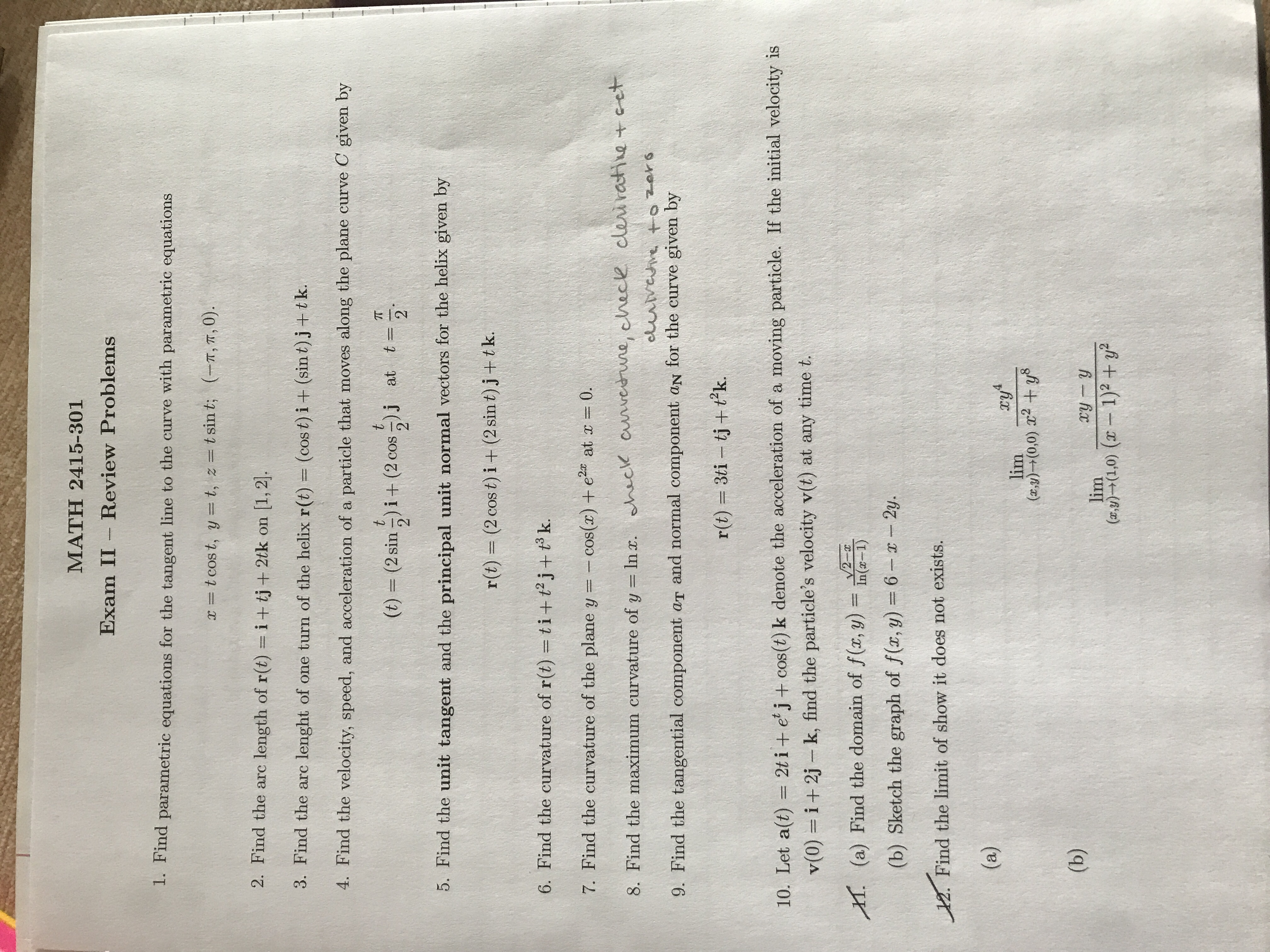MATH 2415-301Exam II Review Problems1. Find parametric equations for the tangent line to the curve with parametric equationst cost, y t, z = t sin t; (-T, T, 0).2. Find the arc length of r(t) = i+ tj+ 2tk on[1,23. Find the arclenght of one turn of the helix r(t) = (cos t) i + (sin t)j+t k.4. Find the velocity, speed, and acceleration of a particle that moves along the plane curve C given byttTTat t=2(t) (2 sin)i+ (2 cos )j5. Find the unit tangent and the principal unit normal vectors for the helix given by(2 cos t) i + (2 sin t)j+tk.r(t)6. Find the curvature of r(t) = ti+ tj+ tk.7. Find the curvature of the plane y = -cos(r)+ eat r = 0.of y In . ack auveerwne, check cleiratie cet8. Find the maximum curvatureeuvevetozars9. Find the tangential component aT and normal component aN for the curve given byr(t) 3ti tj t?k.10. Let a(t) = 2t i+ e j+cos(t) k denote the acceleration of a moving particle. If the initial velocity isi+2j k, find the particle's velocity v(t) at any time t.v(0)2-In(x-1)(a) Find the domain of f (x, y)T(b) Sketch the graph of f(x, y) = 6--2y.12. Find the limit of show it does not exists.(a)4lim()+(0,0) 2 +y8(b)xy ylim(xy)(1,0) (1)2 +y

Question

Help in #1 please.help_outlineImage TranscriptioncloseMATH 2415-301 Exam II Review Problems 1. Find parametric equations for the tangent line to the curve with parametric equations t cost, y t, z = t sin t; (-T, T, 0). 2. Find the arc length of r(t) = i+ tj+ 2tk on [1,2 3. Find the arc lenght of one turn of the helix r(t) = (cos t) i + (sin t)j+t k. 4. Find the velocity, speed, and acceleration of a particle that moves along the plane curve C given by t t TT at t= 2 (t) (2 sin)i+ (2 cos )j 5. Find the unit tangent and the principal unit normal vectors for the helix given by (2 cos t) i + (2 sin t)j+tk. r(t) 6. Find the curvature of r(t) = ti+ tj+ tk. 7. Find the curvature of the plane y = -cos(r)+ e at r = 0. of y In . ack auveerwne, check cleiratie cet 8. Find the maximum curvature euvevetozars 9. Find the tangential component aT and normal component aN for the curve given by r(t) 3ti tj t?k. 10. Let a(t) = 2t i+ e j+cos(t) k denote the acceleration of a moving particle. If the initial velocity is i+2j k, find the particle's velocity v(t) at any time t. v(0) 2- In(x-1) (a) Find the domain of f (x, y) T (b) Sketch the graph of f(x, y) = 6--2y. 12. Find the limit of show it does not exists. (a) 4 lim ()+(0,0) 2 +y8 (b) xy y lim (xy)(1,0) ( 1)2 +y fullscreen
Step 1

Given information:

The parametric equation is

Step 2

Calculation:

'Here,

Step 3

The parameter corresponding to the point (...

Want to see the full answer?

See Solution

Want to see this answer and more?

Our solutions are written by experts, many with advanced degrees, and available 24/7

See Solution
Tagged in

Calculus